# Saturation-Free Numerical Scheme for Computing the Flow Past a Lattice of Airfoils with a Sharp Edge

2019, Vol. 15, no. 2, pp.  135-143

Author(s): Petrov A. G.

The Joukowski – Chaplygin condition, which allows us to determine the circulation of the flow past contour with a sharp edge, is one of the most important achievements of S. A.Chaplygin, whose 150 birthday is celebrated this year. This research is devoted to this problem.
We consider the flow past of a lattice of airfoils by a potential fluid flow. The profile line is determined parametrically by an equation in the form of two dependences of the Cartesian coordinates on the parameter. For a smooth closed loop the Cartesian coordinates are periodic analytical functions. Their Fourier series coefficients decrease exponentially depending on the harmonic number. In the meantime, for a sharp edge loop they decrease much slower — inversely to the square of the harmonic number. Using the symmetric continuation of the profile with a sharp edge, a method of presenting it as a Fourier series with exponentially decreasing coefficients is proposed. Based on this idea, a quickly converging numerical scheme for the computation of the flow past airfoils lattice with a sharp edge by a potential fluid flow has been developed.
The problem is reduced to a linear integro-differential equation on the lattice contour, and then, using specially developed quadrature formulas, is approximated by a linear system of equations. The quadrature formulas converge exponentially with respect to the number of points on the profile and can be rather simply expressed analytically.
Thanks to its quick convergence and high accuracy, this method allows one to optimize profiles by using a direct method by any given integral characteristics. We can find the distribution of the shear stress and the breakaway point on the calculated velocity distribution on the profile from the solution of the boundary layer equation. In the method suggested we do not need to perform the difficult work of constructing the lattice. Also, the problem of scheme viscosity at high Reynolds numbers is omitted.
Keywords: lattice of airfoils, potential fluid flow, sharp edge loop
Citation: Petrov A. G., Saturation-Free Numerical Scheme for Computing the Flow Past a Lattice of Airfoils with a Sharp Edge, Rus. J. Nonlin. Dyn., 2019, Vol. 15, no. 2, pp.  135-143
DOI:10.20537/nd190203

## References

 Weinig, F., Die Strömung um die Schaufeln von Turbomaschinen: Beitrag zur Theorie axial durchströmter Turbomaschinen, Barth, Leipzig, 1935, 142 pp.
 Kochin, N. E., Hydrodynamics Lattice Theory, Gostekhizdat, Moscow, 1949, 103 pp. (Russian)
 Sedov, L. I., Two Dimensional Problems in Hydrodynamics and Aerodynamics, Wiley, New York, 1965, 427 pp.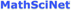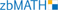Kochin, N. E., Kibel, I. A., and Roze, N. V., Theoretical Hydrodynamics, Wiley, New York, 1964, 583 pp.Stepanov, G. Yu., Hydrodynamics of Turbomachine Lattices, Fizmatlit, Moscow, 1962, 512 pp. (Russian)
 Gostelow, J. P., Cascade Aerodynamics, Pergamon, Oxford, 1984, 270 pp.
 Petrov, A. G., “Saturation-Free Numerical Scheme for Computing the Flow past a Lattice of Airfoils and the Determination of Separation Points in a Viscous Fluid”, Comput. Math. Math. Phys., 51:7 (2011), 1239–1250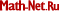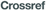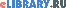; Zh. Vychisl. Mat. Mat. Fiz., 51:7 (2011), 1326–1338 (Russian)Petrov, A. G., “Quadrature Formulas for Periodic Functions and Their Application to the Boundary Element Method”, Comput. Math. Math. Phys., 48:8 (2008), 1266–1283; Zh. Vychisl. Mat. Mat. Fiz., 48:8 (2008), 1344–1361 (Russian)Mamaev, I. S. and Vetchanin, E. V., “The Self-Propulsion of a Foil with a Sharp Edge in a Viscous Fluid under the Action of a Periodically Oscillating Rotor”, Regul. Chaotic Dyn., 23:7–8 (2018), 875–886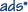Mamaev, I. S., Tenenev, V. A., and Vetchanin, E. V., “Dynamics of a Body with a Sharp Edge in a Viscous Fluid”, Rus. J. Nonlin. Dyn., 14:4 (2018), 473–494Mason, R. J., Fluid Locomotion and Trajectory Planning for Shape-Changing Robots, PhD Dissertation, California Institute of Technology, Pasadena, Calif., 2003, 264 pp.
 Tallapragada, P., “A Swimming Robot with an Internal Rotor As a Nonholonomic System”, Proc. of the American Control Conf. (ACC, Chicago, Ill., July 2015), 657–662